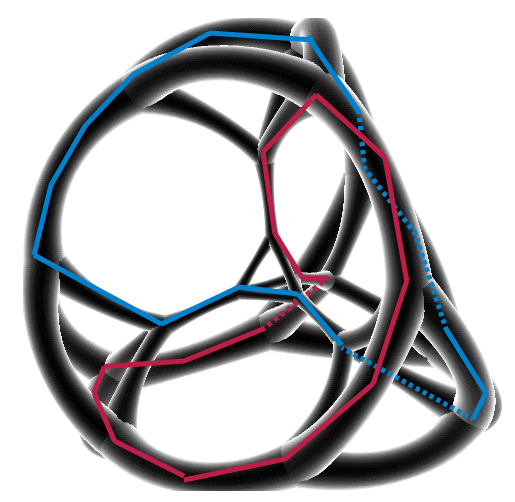The surface of a three-dimensional polyhedron is a two-dimensional space that's topologically equivalent to the sphere. By the Jordan curve theorem, every cycle of edges and vertices in this space cuts the surface into two topological disks. But the surface of a four-dimensional polytope is a three-dimensional space that's topologically equivalent to the hypersphere, or to three-dimensional Euclidean space completed by adding one point at infinity. So, just as in conventional Euclidean space, polygonal chains (such as the cycles of edges and vertices of the polytope) can be nontrivially knotted or linked. If so, this can also be seen in three-dimensions, as a knot or link in the Schlegel diagram of the polytope (a subdivision of a convex polyhedron into smaller convex polyhedra). Does this happen for actual 4-polytopes? Yes! Actually, it's pretty ubiquitous among them.

The linked 4-polytope with the fewest vertices is a duopyramid formed from the convex hull of two equilateral triangles centered at the origin, one in the xy-plane and the other in the zw-plane. These two triangles are not actually two-dimensional faces of the duopyramid; instead, in the Schlegel diagram, they appear as two linked triangles. This polytope has nine tetrahedral facets; in the Schlegel diagram, they appear as one outer tetrahedron, two more adjacent to the top edge of the top linked triangle, two adjacent to the bottom edge of the bottom linked triangle, and four wrapping around the middle vertical edge connecting the two links.Similarly, a seven-vertex neighborly polytope forms a complete graph on seven vertices. As with every embedding of the seven-vertex complete graph into space, it contains a knot.

What about simple 4-polytopes? This means that every vertex has exactly four neighbors. The duopyramid doesn't have this property: its vertices all have five neighbors. The simple 4-polytope with the fewest vertices and facets in which I've found a link is a hypercube, with eight cubical facets.It is also possible to form a trefoil knot in the (4,6)-duoprism, the Cartesian product of a square and a hexagon, and to form a link in the (3,6)-duoprism. These are simple polytopes with ten and nine facets respectively.

There are at least two interesting classes of 4-polytopes that don't have nontrivial knots or links, however. One of these is the class of polyhedral pyramids: 4-dimensional pyramids with a 3-dimensional polyhedron base. Their graphs are apex graphs, embedded nicely with no knots; they have Schlegel diagrams in which the base forms the outside face and the apex of the pyramid is the only vertex inside it, connected to all the other vertices. So any system of closed curves must stay on the planar surface of the base with the exception of one pair of edges through the apex; that's not enough to make a knot or link.

The other is the class of stacked polytopes, formed by gluing simplices face-to-face. Their Schlegel diagrams are formed by repeatedly subdividing a tetrahedron into four smaller tetrahedra meeting at an interior point of the larger tetrahedron, and their graphs are the 4-trees. For any collection of vertex-edge cycles in such a polytope, it's possible to undo one of the subdivision steps and either simplify the collection without changing its topological type, by shortcutting the subdivision vertex, or remove a cycle that forms a face of the polytope. So by induction there can be no knots.

So here's the drawing I was using to figure this out, by overlaying two colored cycles onto the stereographic projection of the truncated 5-cell (same topological embedding into 3d as the Schlegel diagram) from https://commons.wikimedia.org/wiki/File:Truncated_simplex_stereographic.png :